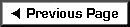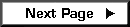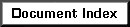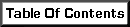A brief digression into material properties is necessary to illustrate how they behave under load. Two aspects are to be considered. First, for a specified sample of a material (say 3" x 3" x 6" tall), how much load can I apply to it before it fails? Second, when the loads are applied, how much is that material going to deform?

Consider the following common construction materials: Styrofoam, wood, concrete, and steel. Let's assume we have samples of each of these materials and we can test them under increasing compression loads. Following each test we remove the load and measure the sample to see if the material has experienced a permanent change in length (also called "deformation").

 The Testing Apparatus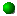In this machine a piston is forced down on top of samples to be tested.  Guide rods are located at the corners so the load is applied uniformly over the top of sample.  Make note of the letter notations as we will use them very soon.

Here are some example results:

1. Styrofoam - Under even small loads (50 lbs.) the cube crushed and did not return to its original shape following removal of load.

2. Wood - Material was loaded in 1,000 pound increments with proportional deformation up to approximately 9000 pounds before permanent deformation was noted. Material resisted higher loading with an increase in deformation until the sample shattered.

3. Concrete - Material was loaded in 1,000 pound increments up to approximately 18,000 pounds before cracking in sample was noted. Deformation was proportional to load until cracking was noted, then increased markedly. When sample failed it seemed to explode and nearly took Joe's head off with one of the chunks.

4. Steel - Material was loaded in 5,000 pound increments up to the capacity of the testing device (100,000 pounds). Deformation was proportional to load in all instances. Larger testing device was not available.

The following graph schematically illustrates the data collected in the previous experiments:

 Results of testing various materialsOf note here is that for three out of four of the materials, there exists a range of loads wherein the deformation is proportional to the applied load. Beyond these ranges, the behavior of the materials was erratic. The wood crushed, the concrete shattered and, if we had been able to demonstrate it, the steel would have squashed.

Graphs like this allow us to predict the behavior of materials. If we know the amount of load we wish to place on a piece of material, we can determine the expected amount of deformation by using our graph. Conversely, we can determine the current load on a piece of material by measuring the deformation and looking up the corresponding load on our graph. Also note that the stronger a material is, the higher the angle of the graphed line.

Expressed in the abstract, the load on a sample of material is the total load "P" (in pounds) divided by the area "A" (in square inches) on which it is applied. The deformation is the change in length "delta" (in inches - sometimes indicated by a small triangle) divided by the original length "L". The slope angle of the line is set by the ratio…

(P/A) / (delta/L)

Rearranged, the slope is…

(P*L)/(A*delta)

This ratio is called the Modulus of Elasticity and has been calculated for almost all materials currently used in construction. The following are examples:

 Cold-rolled Steel 29,500,000 Stainless Steel 27,600,000 Copper 15,600,000 Aluminum Alloys 9,900,000 to 10,300,000 Granite 7,300,000 Concrete 57000 * SQRT(Compressive Strength) = 3,122,000 for 3,000 p.s.i. concrete Common Brick 2,000,000 Douglas Fir - Larch 1,300,000 to 1,900,000

From a practical point of view, there are two ranges for each material: one in which its behavior can be predicted and one in which it is uncertain. It is therefore desirable to design our structures so the materials resist forces (loads) and deflect (deform) in the predictable ranges of their component materials. To prevent us from using materials in the upper regions of their strength capacity, safety factors and other limits agreed to and/or advanced by industry organizations, material institutes and code agencies are applied. And finally, you as the designer apply your own safety factors to the system by your selection and combination of materials.

Now back to shear walls…